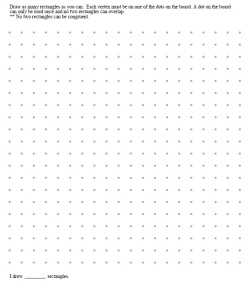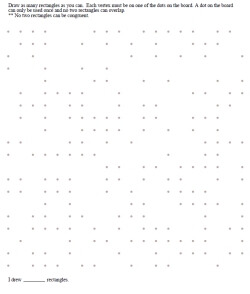edHelper.com Math Critical Thinking Geometry Drawing Shapes Challenge Lesson - Dot Game - Printable Activity Lesson Plan and Worksheets Drawing Shapes Challenge with No Congruent ShapesWorksheets, Lessons, and Printables

Drawing Shapes Challenge: No Two Drawn Shapes Can be Congruent - Draw as many as you canDraw as many different rectangles as you can Draw as many different squares as you can Draw as many different triangles as you can Draw as many as you can - a shape is given - no two shapes drawn can be congruent (trapezoid, parallelogram, pentagon, hexagon, or octagon) Draw as many as you can - a shape is given - no two shapes drawn can be congruent (shape randomly picked)

Half of the Dots are MissingDraw as many different rectangles as you can Draw as many different squares as you can Draw as many different triangles as you can Draw as many as you can - a shape is given - no two shapes drawn can be congruent (trapezoid, parallelogram, pentagon, hexagon, or octagon) Draw as many as you can - a shape is given - no two shapes drawn can be congruent (shape randomly picked)

Drawing Shapes Challenge

Have a suggestion or would like to leave feedback?